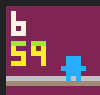an endless platformer in 547 characters of code. (less than 2 tweets!)

has sound, too!

### controls:

• move: arrow keys
• jump: X
• restart: ctrl-r
• mute: ctrl-m
• pause: p / enter

### code:

```poke(24415,16)j=0r=rnd::t::p=1x=64y=0k=-6z=0u=0v=0l={}s=0d=10w=127a=add::_::cls(2)while d<y+99do
d+=16k+=1j=max(j,k)s+=.1m=r(99)for i=0,s do
n=r(w)
if(n>m+16or m>n)a(l,{n,d-2,n,d,8})end
a(l,{0,d+1,m,d,6})a(l,{m+16,d+1,w,d,6})end
v=v*.85+.45if(btnp(5)and g>0)g=0v=-4
u=u*.7-(1+btn()&3)%3+1x=(x+u)%w
y+=v
poke4(24432+r(16),r())z=max(z+s/9,y-90)for i in all(l)do
b,h,e,f,c=unpack(i)
if(h<=y+2and y<=f+2and b<=x+2and x<=e+2)y-=1v=0g=1p=c~=8and y>z
rect(b,h-z,e,f-z,c)end
?k.."\n\fa"..j,1,1,7
?"\fc웃\^1",x-3,y-3-z\1
if(p)goto _
?"\ag3e-c"
?"\^5"
goto t```
 Status Released Platforms HTML5 Author pancelor Genre Action, Platformer Made with PICO-8, Sublime Text Tags 2D, PICO-8, Short, sourcecode Average session A few seconds Inputs Keyboard, Gamepad (any), Touchscreen Accessibility Color-blind friendly, High-contrast, Textless

## Development log

(1 edit) (+1)

This is surprisingly good, nice job. It feels like a lost Atari game and really gets you in a "just one more try" mood. My best score is 65.

(1 edit) (+1)

wowowow that's a "game-ass-game," right there

great prioritization in your feature set to keep inside the char limit, super nice job on this!!

59 is my best so far, but somebody can surely trounce that